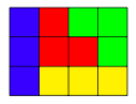시간 제한메모리 제한제출정답맞힌 사람정답 비율
2 초 512 MB253924127.333%

## 문제

We have a grid of height $3$ and width $n$, as well as pieces that occupy $3$ adjacent cells. Given $n$, determine the number of ways to fill the grid so that each cell is covered by exactly one piece and no piece sticks out of the grid. Here there is an example of a way to fill a grid of width $4$:Notice that any piece will be a rotation of one of the pieces of this example. Find the answers modulo $10^9 + 7$.

## 입력

The first line contains an integer $t$, the number of test cases ($1 \leq t \leq 100$).

Each test case is given on a separate line containing an integer $n$ ($1 \leq n \leq 10^{18}$), the width of the grid.

## 출력

For each test case, print a line with a single integer: the number of ways to fill the grid with aforementioned conditions modulo $10^9 + 7$.

## 예제 입력 1

3
1
2
3


## 예제 출력 1

1
3
10